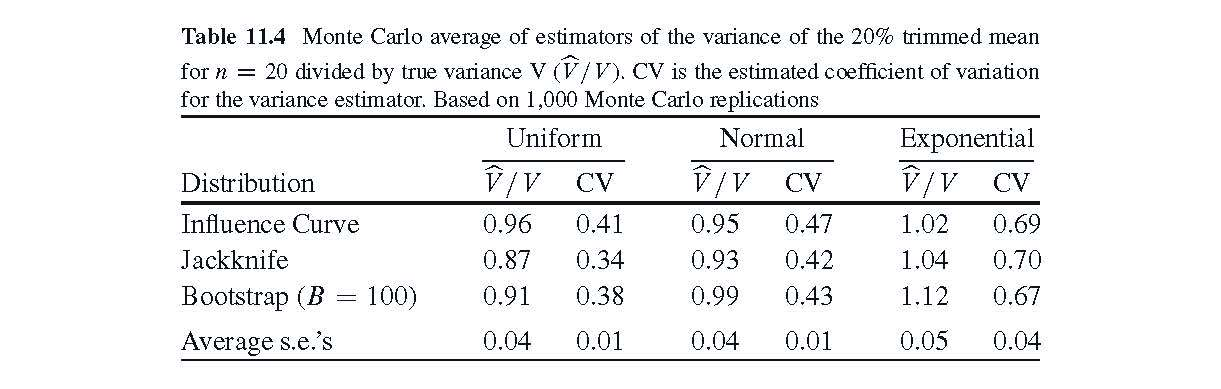# Monte Carlo Standard Errors for Summary Statistics Based on Multiple Columns of Simulation Output

#### 2019-05-01

In this vignette, we reproduce a piece of Table 11.4, p. 423, of Boos and Stefanski (2013), “Essential Statistical Inference.” Our goal is to illustrate the use of mc.se.matrix to get the average standard errors (SEs) for sets of table entries (see the last row of the following table).Table 11.4 compares three methods of estimating the variance of the 20% trimmed mean. The three methods are the Influence Curve Method (Delta Method), the jackknife, and the bootstrap. The main entries of the table are based on N Monte Carlo samples of size n=20, leading to a matrix of N by 3 variance estimators, each column for a different method. The main entries in Table 11.4 are

$$(\widehat{V}/V)$$ = the average of N sample-by-sample variance estimators $$(\widehat{V}$$) divided by the sample variance of the N estimators ($$V$$), and

CV = the coefficient of variation of the N variance estimators

If a variance estimator is approximately unbiased for the true variance, then $$(\widehat{V}/V)$$ will be close to 1.0. Coupled with a SE, $$(\widehat{V}/V)$$ is very easy to quickly assess and compare biases. The CV of a variance estimator is useful for comparing variance estimators in terms of a comparable measure of variability. Mean Squared Error (MSE) is not as useful for comparing variance estimators because it tends to favor estimators that are biased low (since the variance goes down with the mean).

To create Table 11.4, we need 12 columns of Monte Carlo output for the trimmed mean, the 3 variance estimators, and 3 distributions ((1+3)*3=12). For simplicity, we just work with the 4 columns that produced the results for the Uniform(0,1) distribution. Here are the steps.

1. Get code for the Influence Curve variance estimator of the 20% trimmed mean. The code for the jackknife and bootstrap are already part of this package.
trim20 <- function(x){mean(x,.2)} # 20% trimmed mean function
trim.var <- function(x,trim){     # var. est. for trim20
n <- length(x);h<-floor(trim*n)
tm <- mean(x,trim);sort(x)->x
ss <- h*((x[h+1]-tm)^2+(x[n-h]-tm)^2)
ss <- ss+sum((x[(h+1):(n-h)]-tm)^2)
ss/((n-2*h)*(n-2*h-1))
}
trim20.var<-function(x){trim.var(x,.2)}
1. Create the N=1,000 datasets of size n=20, and then use apply to get the N variance estimators for each method and put them in the output matrix hold.
hold <- matrix(0,nrow=1000,ncol=4)
set.seed(351)
z <- matrix(runif(1000*20),nrow=1000)
hold[,1] <- apply(z,1,trim20)
hold[,2] <- apply(z,1,trim20.var)
hold[,3] <- apply(z,1,jack.var,theta=trim20)
hold[,4] <- apply(z,1,boot.var,theta=trim20,B=100)
1. To Create the table entries and associated SEs, we use the following auxiliary functions. A key point is to notice that ratio.mean.vhat.var is a function with two arguments, the first is for indexing the rows of the data in the second argument xdata. The reason for this construction is that jackknife SEs require us to leave out rows of the data matrix. The CV entries of the table do not need this special construction because they are based only on one column of the data matrix.
ratio.mean.vhat.var <- function(index,xdata){# estimates in col 1, vhats in col. 2
mean(xdata[index,2])/var(xdata[index,1])}

cv <- function(x){sd(x)/mean(x)}

Then for the $$(\widehat{V}/V)$$ entries

mc.se.matrix(x=hold,xcol=c(1,2),summary.f=ratio.mean.vhat.var)
summary        se    N    method
1 0.9561514 0.0443825 1000 Jackknife

mc.se.matrix(x=hold,xcol=c(1,3),summary.f=ratio.mean.vhat.var)
summary         se    N    method
1 0.8714109 0.03963418 1000 Jackknife

mc.se.matrix(x=hold,xcol=c(1,4),summary.f=ratio.mean.vhat.var)
summary         se    N    method
1 0.9093518 0.04170819 1000 Jackknife

and the column summary of SEs

> mean(0.0443825,0.03963418,0.04170819)
 0.0443825

Since CV only involves one column of hold, we now use mc.se.vector.

> mc.se.vector(x=hold[,2],summary.f=cv)
summary         se    N    method
1 0.4071375 0.00876722 1000 Jackknife

> mc.se.vector(x=hold[,3],summary.f=cv)
summary          se    N    method
1 0.3389507 0.006995271 1000 Jackknife

> mc.se.vector(x=hold[,4],summary.f=cv)
summary          se    N    method
1 0.3687566 0.008063226 1000 Jackknife

And the mean of the CV SEs is

> mean(0.00876722,0.006995271,0.008063226)
 0.00876722

Note that the summary pieces of the above output are the main entries in Table 11.4 after rounding.